# Factoring a number and prime numbers?

A number's factors are numbers which multiply together to form it as a product. Another way of thinking of this is that every number is the product of multiple factors. Learning how to factor - that is, break up a number into its component factors - is an important mathematical skill that is used not only in basic arithmetic but also in algebra, calculus, and beyond

A number can be factored into a product of its component factors
A number can be factored into a product of its prime .
But, What exactly is a prime number ?
Prime numbers are numbers greater than 1 that are evenly divisible only by themselves and 1

Is it a number that can only be evenly divided by that number itself and one ?As in like it cannot be factored further?

Last edited:

Yes, if one could factor primes further then they would be divisible by another number and hence wouldn't be primes. In fact beyond that every number can be uniquely factored into its components, which is one of the fundamental theorems of number theory.

Ok , Thanks . so its a bit like this ?

Prime Numbers

The first few prime numbers are 2, 3, 5, 7, 11, 13 .
A prime number is a positive integer which has no factors other than 1 and itself. 1 itself, by definition, is not a prime number.
Prime numbers cant be divided any further and thus can be thought of as the atoms of numbers.
Any number which is not prime can be written as the product of prime numbers, we simply keep dividing it into more parts until all factors are prime

84 = 2 x 2 x 3 x 7

?

Yes and as I mentioned above every number can be factored into its prime factors in one way only. This is also the reason why 1 is not condsidered a prime number.

Thanks a lot

I am not sure where to from here , can i move to algebra and practice some problems ?

Does algebra involve prime factorization ?

Last edited:
Does algebra involve prime factorization ?
No, I do not think so. I believe that prime factorization is part of pre-algebra and number theory. The only connection I can think of is factorizing polynomials, which may involve thinking about the factors of a number.
I am not sure where to from here , can i move to algebra and practice some problems ?
I am not sure which type of questions you are looking for. If you are looking for questions, which involve simply factorizing numbers I would recommend a website called " Brilliliant.org". They offer problems and information on nearly all branches of maths and offer those kinds of practice problems.

Sometimes i don't even know what to search for .

Thanks a lot

fresh_42
Mentor
A number can be factored into a product of its component factors
"Component factors" isn't a well-defined term. The correct term would be "irreducible factors" to emphasize that those cannot be split further. The others are called reducible.
A number can be factored into a product of its prime .
This is a theorem, which holds in some kind of number sets and which says: irreducible numbers are prime numbers. It is not automatically true, e.g. the real numbers don't have any primes.
But, What exactly is a prime number ?
A number is called prime, if whenever it divides a product, it has to divide (at least) one of the factors: ##p\,\vert \,a\cdot b \Longrightarrow p\,\vert \,a \, \vee \, p\,\vert \,b##.
This means that primes are always irreducible but not necessarily all irreducible elements are prime.
Prime numbers are numbers greater than 1 that are evenly divisible only by themselves and 1
Yes, because it can be shown that irreducible and prime are the same in the integers. The fact that unities like ##\pm 1## are ruled out is deliberately made. The purpose to do so is to formulate theorems in a reasonable way, since one can always add arbitrary many ones or evenly many minus ones to a factorization without changing the result. If unities (elements with a multiplicative inverse) were allowed, it would be harder to get to the core meaning of prime numbers or unnecessarily complicated to formulate results: "Let ##p## be a prime, but not a unity, then ..." would have been to added almost everywhere.
Is it a number that can only be evenly divided by that number itself and one ?As in like it cannot be factored further?

Last edited:
•QuantumQuest
I am a bit confused ,i was trying to learn polynomial factorization . but before that , i thought i would refresh my arithmetic factorization skills . these things are confusing me a lot .

Especially terms like , factors , prime factors ,factorize , factorization

number factors.
algebraic factors

not sure which term belongs to which or what term should be used where ...

If you write a polynomial as the product of two or more polynomials, you have factored the polynomial.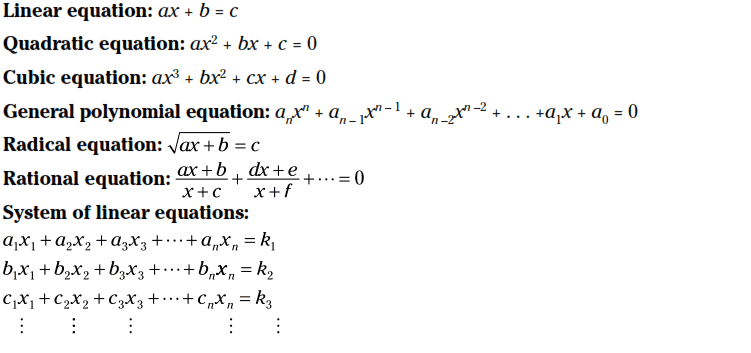Here is an example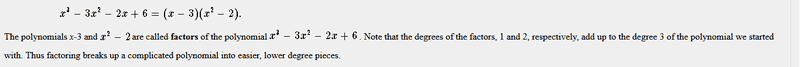how do i factor a polynomial ?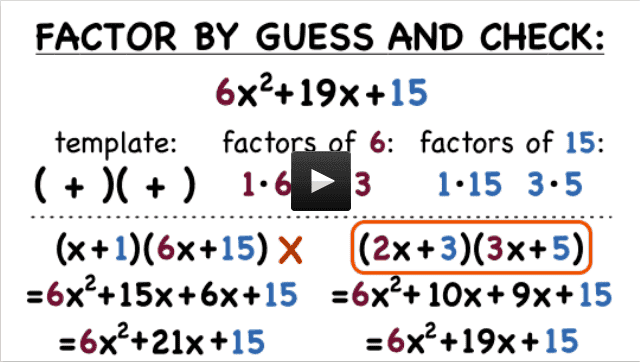The steps involved ...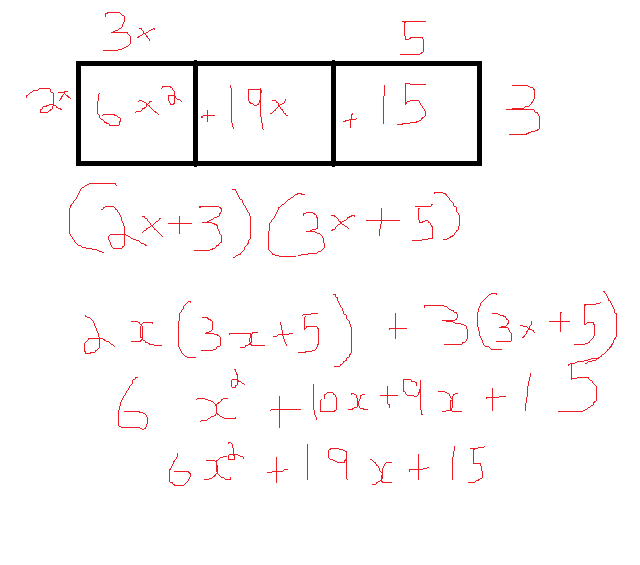anyway what my main aim is to learn about polynomial factorization itself , i hope that's what it is actually called ... :)

Mark44
Mentor
I am a bit confused ,i was trying to learn polynomial factorization . but before that , i thought i would refresh my arithmetic factorization skills . these things are confusing me a lot .

Especially terms like , factors , prime factors ,factorize , factorization

number factors.
algebraic factors

not sure which term belongs to which or what term should be used where ...
factors -- expressions that can be multiplied to produce some given expression. For example, 4 and 9 are factors of 36, because 4 * 9 = 36. Also 2x and 5x are factors of 10x2, because (2x)(5x) = 10x2.
prime factors - expressions that can't be further broken up into simpler factors. For example, the prime factors of 36 are 2, 2, 3, and 3, since 2 * 2 * 3 * 3 = 36. 4 and 9 are factors of 36, but they aren't prime factors. Both 2 and 3 are prime numbers (or primes). A number (integer) is prime if its only factors are 1 and the number itself.
factorize (or factor) - (verb) to reduce an expression into a product (multiplication) of subexpressions. In the US we typically say "factor an expression" rather than "factorize an expression." This shorthand usage might be confusing to some, because "factor" can be used as both a noun (e.g., "7 is a factor of 49") and as a verb (e.g., "factor 15 into a product of prime numbers.").
In your previous post you factored the trinomial ##6x^2 + 19x + 16## into a product of two binomial factors ##(2x + 3)(3x + 5)##.
factorization - (noun) the action of reducing an expression into a product of its factors.

•QuantumQuest
Thanks a lot for explaining a lot of things Mark44 , it nice to most of the important points in one thread :)

Thanks , I am sticking with the simple ones , that stuff looks way too complicated :)

A little bit of an update is ok i guess ... This started with a lot of confusions about arithmetic and algebra .
I thought i would share some stuffs which i was able to narrow down to for beginners like me ...
I hope i am not going too much off topic here .

i found this really nice website when i was searching for some terms

http://www.mathhands.com/

whole numbers , natural numbers , integers , rational numbers , irrational numbers .

factors ( reducible), prime factors (irreducible), greatest common factor (same as greatest common divisor ), least common multiple

Monomial , binomial , trinomial , polynomial

simplify ,factor , factoring (factorization )

’To factor’ means to break up into multiples

Factor by Distributive law method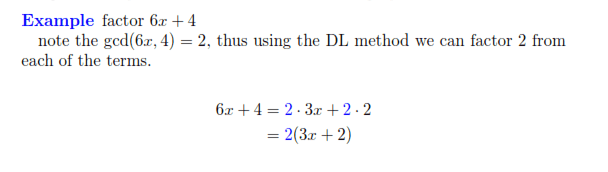Factor by grouping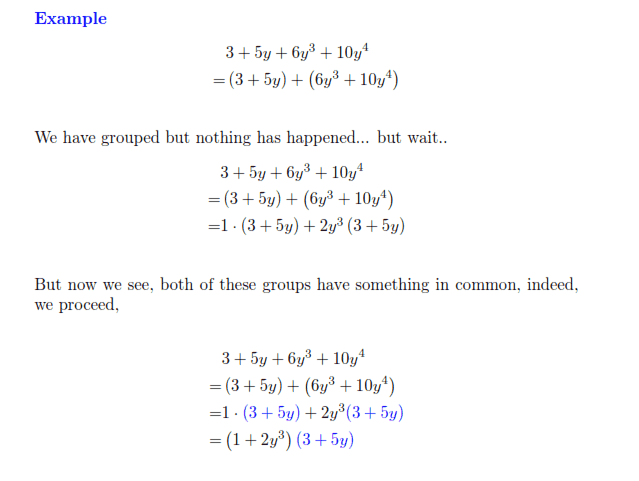Factor by Splitting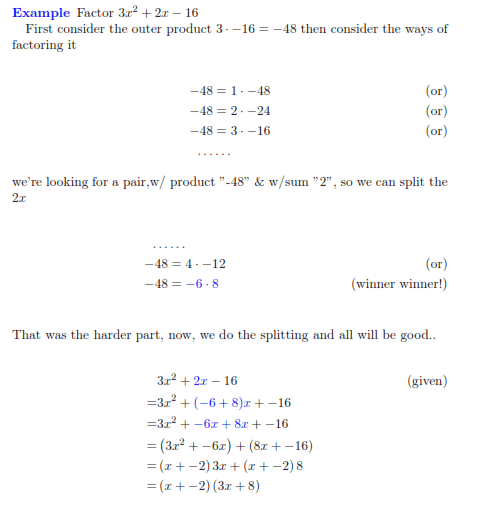Factor by Very Famous Polynomials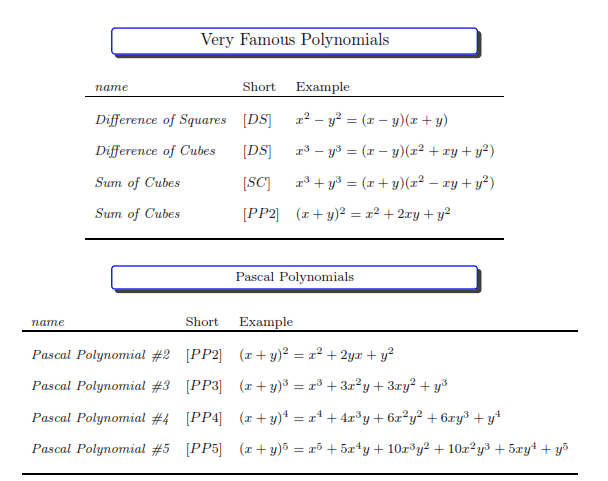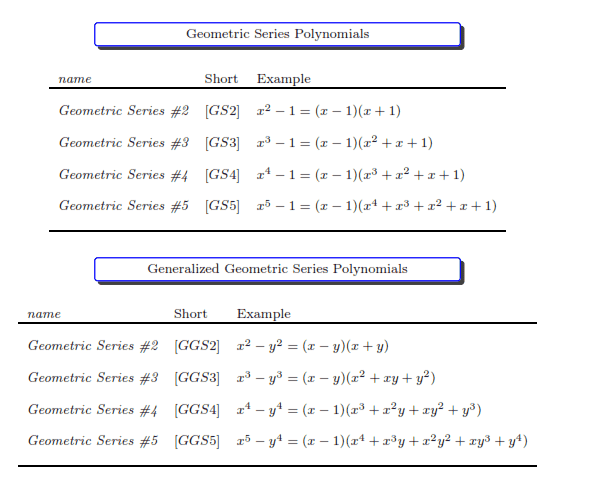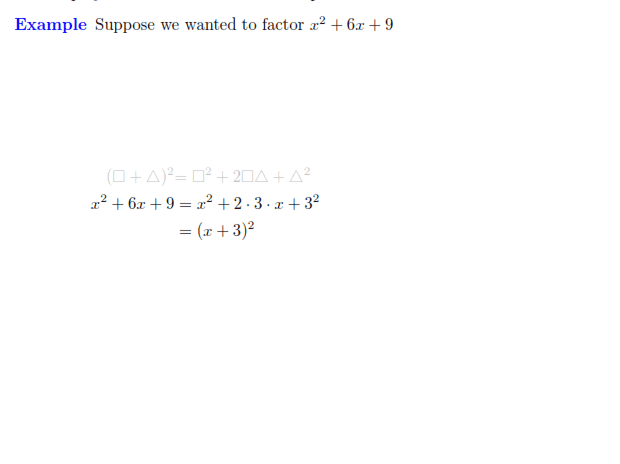Not sure ,where to from here ?

Last edited:
fresh_42
Mentor
Not sure ,where to from here ?
What do you mean by "where to"?

Is that all , or are there more methods ?

Mark44
Mentor
Not sure ,where to from here ?
What do you mean by "where to"?
Probably means "where to go from here".

@rosiekidcute, you posted images of all of those factoring examples. If you understand them all and are able to work examples of them correctly, then you're in pretty good shape, and should continue on with other topics. As I recall, you are reviewing topics in algebra from a book you purchased. I would suggest just continuing to work through your book. Possible topics would include working with various types of equations, such as equations with radicals, equations with rational expressions, equations with absolute values, linear and quadratic inequalities, graphs of linear functions, quadratic functions, and so on.

fresh_42
Mentor
Probably means "where to go from here".
Yes. I simply saw no open question.

Yes. I simply saw no open question.

Yes , that's what i meant . where to go from here ...

Probably means "where to go from here".

@rosiekidcute, you posted images of all of those factoring examples. If you understand them all and are able to work examples of them correctly, then you're in pretty good shape, and should continue on with other topics. As I recall, you are reviewing topics in algebra from a book you purchased. I would suggest just continuing to work through your book. Possible topics would include working with various types of equations, such as equations with radicals, equations with rational expressions, equations with absolute values, linear and quadratic inequalities, graphs of linear functions, quadratic functions, and so on.

Thanks a lot for the advice Mark44 . I will try to work with practice problems from the three books i have with Algebra :)